output.to from Sideway
Draft for Information Only

# Content

`Equilibrium of Rigid Body Free-Body Free-Body Diagram`

# Equilibrium of Rigid Body

A rigid body is in static equilibrium state when the rigid body remains stationary and is not accelerating in any way. In other words, a rigid body is in equilibrium when both the resultant force and the resultant moment are zero for all the forces and couples acting on it. Through system of forces transformation, an equivalent system of forces can be reduced to a force vector and a couple vector. Therefore the resultant force and the resultant couple vectors acting on the rigid body are equal to zero. That is: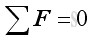and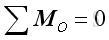Or in terms of rectangular components: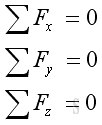and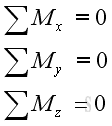In other words,  there is no translational and rotational motion when an object is in static equilibrium state.## Free-Body

In engineering static application, a rigid body is in equilibrium when the systems of external forces cause no translational and rotational motion to the rigid body since the resultant force and resultant memont on the rigid body are zero. For example, a hanging mass from the ceiling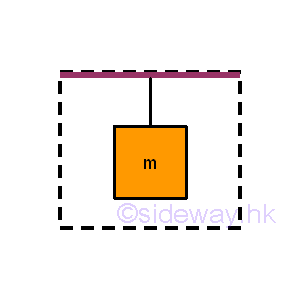When solving the equilibrium problem of the considered body, in order to focus only on the interested body instead of the whole system, the interested body should be isolated from other bodies as a free body. According to Newton's third law, each applied force will have a reaction force of equal in magnitude and opposite in direction acting on the interacting bodies. Therefore, all the surrounding uninterested objects can be stripped away by replacing with the corresponding reaction force acting on the interesed body. For example, the interested objects are the mass and string.Therefore, a free body diagram can be drawn by adding a reaction force to replace the force due to the ceiling, while the body force due to the body mass remains unchange.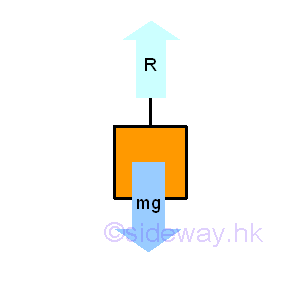Since the hanging string is also not an interested body, Imply the free body diagram of the body mass is :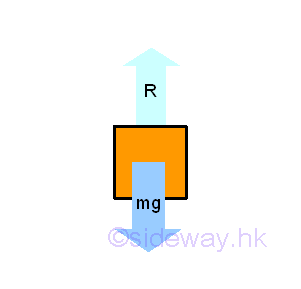## Free-Body Diagram

Important steps of constructing the free-body diagram are:

1. Selecting the interested bodys from the whole system, so that the free body of interest can be detached away from the ground, connections, supports and all other bodies.

2. In general, there are always forces exerted on the free body by those stripped away bodies. These forces are considered as external forces and are represented by forces acting on the free body.

3. Besides, the weight of the free body should also be considered and be included as external forces acting on the free body.

4. But for forces exerted on each other between various parts of the free body, these forces are considered as internal forces because the free body is considered as a rigid body also.

5. For known external forces, such as weight of free body, applied forces, both the magnitudes and directions of each known external forces should be marked at the corresponding position on the free-body diagram.

6. For those unknown external forces due to stripping away bodies, such as ground, connections, supports and all other bodies from the free body, these forces are usually the reaction forces to opposite the motion of the free body in order to constrain the free body to remain in the same static position. Each known external forces should also be marked at the corresponding position on the free-body diagram. Although the magnitudes of unknown external forces are unknown, the reaction directions of each unknown external forces at the supports and connections can usually determined by its type of support or connection.

7. The pointing direction of an unknown external forces after the avaialable reaction are determined can be assumed in any direction because the sense of the direction will confirm whether the assumption is correct or not.

8. Although, the profile of the free body can usually be neglected, the free-body diagram should include dimensions for calculating the moments of forces.
9. Finally, including a coordinate axis is also necessary for determining the sign and angle of forces

ID: 120200059 Last Updated: 2/15/2012 Revision: 0 Ref:References

1. I.C. Jong; B.G. rogers, 1991, Engineering Mechanics: Statics and Dynamics
2. F.P. Beer; E.R. Johnston,Jr.; E.R. Eisenberg, 2004, Vector Mechanics for Engineers: StaticsHome 5

Management

HBR 3

Information

Recreation

Culture

Chinese 1097

English 339

Computer

Hardware 224

Software

Application 213

Latex 52

Manim 204

KB 1

Numeric 19

Programming

Web 289

Unicode 504

HTML 66

CSS 65

SVG 46

ASP.NET 270

OS 429

Python 72

Knowledge

Mathematics

Algebra 84

Geometry 33

Calculus 67

Engineering

Mechanical

Rigid Bodies

Statics 92

Dynamics 37

Control

Natural Sciences

Electric 27